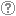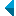# Iskanje po repozitorijuIskalni niz: išči po NaslovAvtorOpisKljučne besedePolno besediloLeto izida INALIIN NE išči po NaslovAvtorOpisKljučne besedePolno besediloLeto izida INALIIN NE išči po NaslovAvtorOpisKljučne besedePolno besediloLeto izida INALIIN NE išči po NaslovAvtorOpisKljučne besedePolno besediloLeto izida Vrsta gradiva: Vse vrste gradiv Habilitacijsko delo (m4) Višješolska diplomska naloga (m6) Specialistično delo (m3) Diplomsko delo * (dip) Magistrsko delo * (mag) Doktorska disertacija * (dok) Raziskovalni podatki ali korpus (data) * po starem in bolonjskem študiju Jezik: Vsi jezikiSlovenski jezikAngleški jezikNemški jezikHrvaški jezikSrbski jezikBolgarski jezikBosanski jezikČeški jezikFinski jezikFrancoski jezikItalijanski jezikJaponski jezikLitvanski jezikMadžarski jezikNemški jezik (Avstrija)Norveški jezikPoljski jezikRuski jezikSlovaški jezikSrbski jezik (cirilica)Španski jezikŠvedski jezikTurški jezikNeznan jezik Išči po: RUP    FAMNIT - Fakulteta za matematiko, naravoslovje in informacijske tehnologije    FHŠ - Fakulteta za humanistične študije    FM - Fakulteta za management    FTŠ Turistica - Fakulteta za turistične študije - Turistica    FVZ - Fakulteta za vede o zdravju    IAM - Inštitut Andrej Marušič    PEF - Pedagoška fakulteta    UPR - Univerza na Primorskem    ZUP - Založba Univerze na PrimorskemCOBISS    Fakulteta za humanistične študije, Koper    Fakulteta za management Koper in Pedagoška fakulteta Koper    Fakulteta za vede o zdravju, Izola    Knjižnica za tehniko, medicino in naravoslovje, Koper    Turistica, Portorož    Znanstveno-raziskovalno središče Koper Opcije: Prikaži samo zadetke s polnim besedilom Ponastavi

 1 - 10 / 142123456789101.Graphs and their automorphism groups bpredavanje eUnidad Cuernavaca del Instituto de Matemáticas, UNAM, Cuernavaca, Morelos, Mehika, 23. - 27. 7. 2012Klavdija Kutnar, Primož Šparl, 2012, predavanje na tuji univerziNajdeno v: osebiKljučne besede: automorphism groupObjavljeno: 15.10.2013; Ogledov: 2653; Prenosov: 34Polno besedilo (0,00 KB) 2.Classification of cubic symmetric tricirculantsKlavdija Kutnar, István Kovács, Dragan Marušič, Steve Wilson, 2012, izvirni znanstveni članekNajdeno v: osebiKljučne besede: symmetric graph, semiregular, tricirculantObjavljeno: 15.10.2013; Ogledov: 2751; Prenosov: 76Polno besedilo (0,00 KB) 3.Hamilton paths in vertex-transitive graphs of order 10pDragan Marušič, Klavdija Kutnar, Cui Zhang, 2012, izvirni znanstveni članekOpis: It is shown that every connected vertex-transitive graph of order ▫$10p$▫, ▫$p \ne 7$▫ a prime, which is not isomorphic to a quasiprimitive graph arising from the action of PSL▫$(2,k)$▫ on cosets of ▫$\mathbb{Z}_k \times \mathbb{Z}_{(k-1)/10}$▫, contains a Hamilton path.Najdeno v: osebiKljučne besede: graph, vertex-transitive, Hamilton cycle, Hamilton path, automorphism groupObjavljeno: 15.10.2013; Ogledov: 2964; Prenosov: 36Polno besedilo (0,00 KB) 4.On prime-valent symmetric bicirculants and Cayley snarksKlavdija Kutnar, Ademir Hujdurović, Dragan Marušič, 2013, objavljeni znanstveni prispevek na konferenciNajdeno v: osebiKljučne besede: graph, Cayley graph, arc-transitive, snark, semiregular automorphism, bicirculantObjavljeno: 15.10.2013; Ogledov: 2728; Prenosov: 149Polno besedilo (0,00 KB) 5.Hamilton cycles and paths in vertex-transitive graphsKlavdija Kutnar, 2011, predavanje na tuji univerziNajdeno v: osebiKljučne besede: Hamilton cyclesObjavljeno: 15.10.2013; Ogledov: 2085; Prenosov: 34Polno besedilo (0,00 KB) 6.Hamiltonicity of vertex-transitive graphs of order 4pKlavdija Kutnar, Dragan Marušič, 2008, izvirni znanstveni članekOpis: It is shown that every connected vertex-transitive graph of order ▫$4p$▫, where ▫$p$▫ is a prime, is hamiltonian with the exception of the Coxeter graph which is known to possess a Hamilton path.Najdeno v: osebiKljučne besede: graph theory, vertex-transitive graphs, Hamilton cycle, automorphism groupObjavljeno: 15.10.2013; Ogledov: 2882; Prenosov: 34Polno besedilo (0,00 KB) 7.Edge-transitive Rose Window graphsKlavdija Kutnar, 2008, predavanje na tuji univerziNajdeno v: osebiObjavljeno: 15.10.2013; Ogledov: 3755; Prenosov: 43Polno besedilo (0,00 KB) 8.Distance-balanced graphs: Symmetry conditionsAleksander Malnič, Klavdija Kutnar, Dragan Marušič, Štefko Miklavič, 2006, izvirni znanstveni članekOpis: A graph ▫$X$▫ is said to be distance-balanced if for any edge ▫$uv$▫ of ▫$X$▫, the number of vertices closer to ▫$u$▫ than to ▫$v$▫ is equal to the number of vertices closer to ▫$v$▫ than to ▫$u$▫. A graph ▫$X$▫ is said to be strongly distance-balanced if for any edge ▫$uv$▫ of ▫$X$▫ and any integer ▫$k$▫, the number of vertices at distance ▫$k$▫ from ▫$u$▫ and at distance ▫$k+1$▫ from ▫$v$▫ is equal to the number of vertices at distance ▫$k+1$▫ from ▫$u$▫ and at distance ▫$k$▫ from ▫$v$▫. Exploring the connection between symmetry properties of graphs and the metric property of being (strongly) distance-balanced is the main theme of this article. That a vertex-transitive graph is necessarily strongly distance-balanced and thus also distance-balanced is an easy observation. With only a slight relaxation of the transitivity condition, the situation changes drastically: there are infinite families of semisymmetric graphs (that is, graphs which are edge-transitive, but not vertex-transitive) which are distance-balanced, but there are also infinite families of semisymmetric graphs which are not distance-balanced. Results on the distance-balanced property in product graphs prove helpful in obtaining these constructions. Finally, a complete classification of strongly distance-balanced graphs is given for the following infinite families of generalized Petersen graphs: GP▫$(n,2)$▫, GP▫$(5k+1,k)$▫, GP▫$(3k 3,k)$▫, and GP▫$(2k+2,k)$▫.Najdeno v: osebiKljučne besede: graph theory, graph, distance-balanced graphs, vertex-transitive, semysimmetric, generalized Petersen graphObjavljeno: 15.10.2013; Ogledov: 3249; Prenosov: 85Polno besedilo (0,00 KB) 9.Hamilton cycles and paths in vertex-transitive graphsKlavdija Kutnar, 2008, predavanje na tuji univerziNajdeno v: osebiObjavljeno: 15.10.2013; Ogledov: 3214; Prenosov: 33Polno besedilo (0,00 KB) 10.A complete classification of cubic symmetric graphs of girth 6Klavdija Kutnar, Dragan Marušič, 2009, izvirni znanstveni članekOpis: A complete classification of cubic symmetric graphs of girth 6 is given. It is shown that with the exception of the Heawood graph, the Moebius-Kantor graph, the Pappus graph, and the Desargues graph, a cubic symmetric graph ▫$X$▫ of girth 6 is a normal Cayley graph of a generalized dihedral group; in particular, (i) ▫$X$▫ is 2-regular if and only if it is isomorphic to a so-called ▫$I_k^n$▫-path, a graph of order either ▫$n^2/2$▫ or ▫$n^2/6$▫, which is characterized by the fact that its quotient relative to a certain semiregular automorphism is a path. (ii) ▫$X$▫ is 1-regular if and only if there exists an integer ▫$r$▫ with prime decomposition ▫$r=3^s p_1^{e_1} \dots p_t^{e_t} > 3$▫, where ▫$s \in \{0,1\}$▫, ▫$t \ge 1$▫, and ▫$p_i \equiv 1 \pmod{3}$▫, such that ▫$X$▫ is isomorphic either to a Cayley graph of a dihedral group ▫$D_{2r}$▫ of order ▫$2r$▫ or ▫$X$▫ is isomorphic to a certain ▫$\ZZ_r$▫-cover of one of the following graphs: the cube ▫$Q_3$▫, the Pappus graph or an ▫$I_k^n(t)$▫-path of order ▫$n^2/2$▫.Najdeno v: osebiKljučne besede: graph theory, cubic graphs, symmetric graphs, ▫$s$▫-regular graphs, girth, consistent cycleObjavljeno: 15.10.2013; Ogledov: 3191; Prenosov: 82Polno besedilo (0,00 KB)
Iskanje izvedeno v 0 sek.
Na vrh